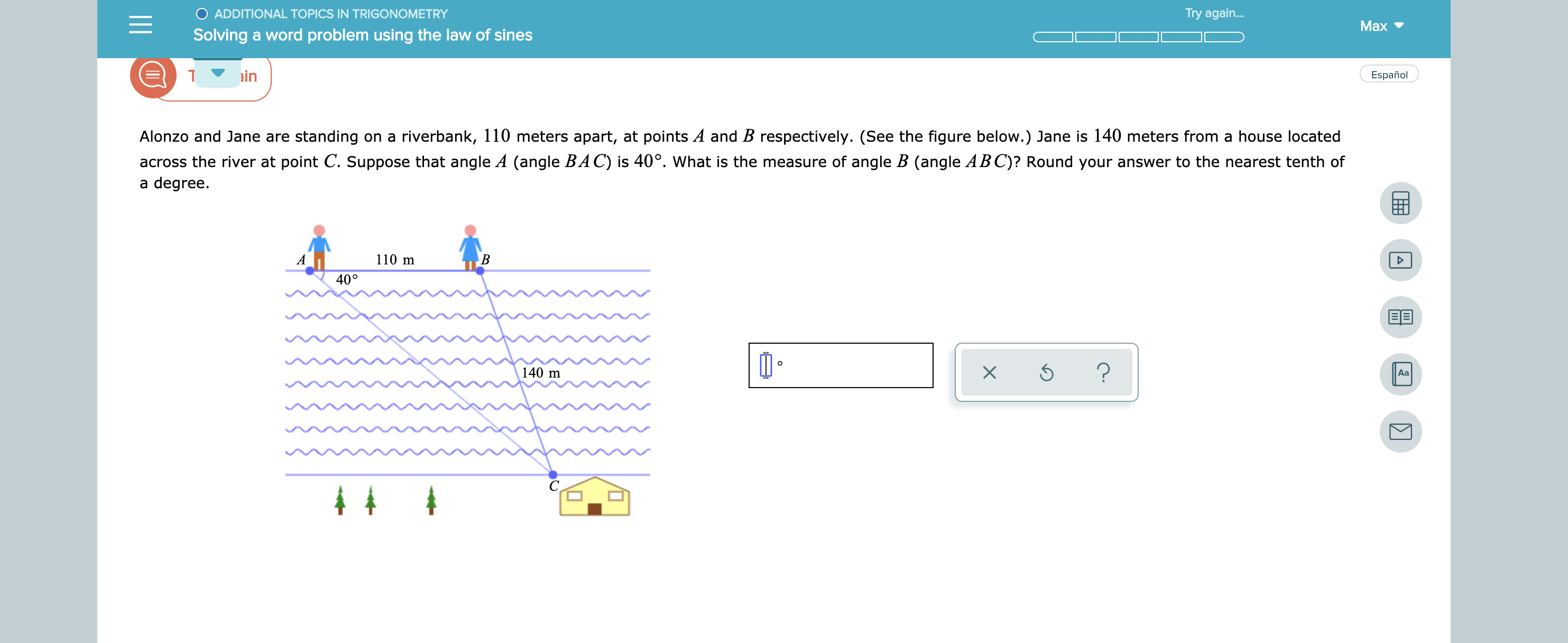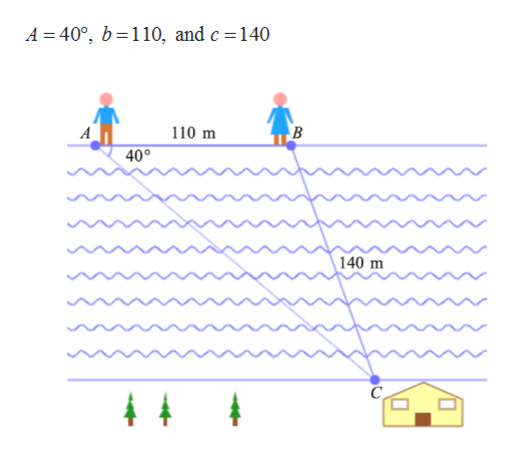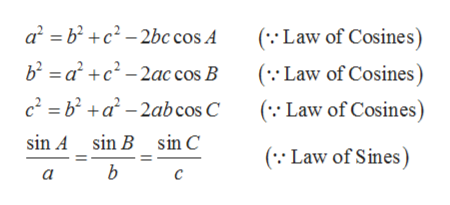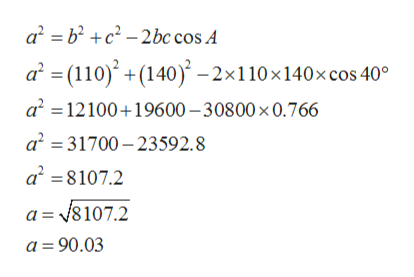O ADDITIONAL TOPICS IN TRIGONOMETRYTry again...MaxSolving a word problem using the law of sinesinEspañolAlonzo and Jane are standing on a riverbank, 110 meters apart, at points A and B respectively. (See the figure below.) Jane is 140 meters from a house locatedacross the river at point C. Suppose that angle A (angle BA C) is 40°. What is the measure of angle B (angle ABC)? Round your answer to the nearest tenth ofa degree.110 mAB40°O?X140 mАаС4 4ш

Question

see attachmenthelp_outlineImage TranscriptioncloseO ADDITIONAL TOPICS IN TRIGONOMETRY Try again... Max Solving a word problem using the law of sines in Español Alonzo and Jane are standing on a riverbank, 110 meters apart, at points A and B respectively. (See the figure below.) Jane is 140 meters from a house located across the river at point C. Suppose that angle A (angle BA C) is 40°. What is the measure of angle B (angle ABC)? Round your answer to the nearest tenth of a degree. 110 m A B 40° O ? X 140 m Аа С 4 4 ш fullscreen
Step 1

Given: A triangle ABC as in the figure below below. Suppose thathelp_outlineImage TranscriptioncloseA 40°, b 110, and c = 140 110 m B 40° 140 m 4 4 fullscreen
Step 2

Formula used:

For any triangle given two sides and an included angle, the Law of Cosines and Law of Sines:help_outlineImage Transcriptionclosea2 bc2-2bc cos A b2 ac-2ac cos B c2 =b2+a-2ab cos C ( Law of Cosines (Law of Cosines ( Law of Cosines) sin A sin B sin C ( Law of Sines) b fullscreen
Step 3

By finding the value of ‘a&r...help_outlineImage Transcriptionclosea b2c2-2bc cos A a (110)(140)-2x110x140x cos 40° a2 12100 19600-30800 x 0.766 a2 31700-23592.8 a28107.2 a =8107.2 a 90.03 fullscreen

Want to see the full answer?

See Solution

Want to see this answer and more?

Our solutions are written by experts, many with advanced degrees, and available 24/7

See Solution
Tagged in

Trigonometry